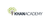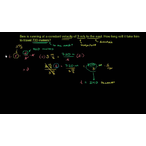Physics: Solving for time

Type product
Tijdsduur

Physics: Solving for timeKhan Academy

Tip: laat jezelf inspireren, bekijk hier de video!Beschrijving

Rate of change in position, or speed, is equal to distance traveled divided by time. To solve for time, divide the distance traveled by the rate. For example, if Cole drives his car 45 km per hour and travels a total of 225 km, then he traveled for 225/45 = 5 hours.

Physics

Learn about the basic principles that govern the physical world around us. Solid understanding of algebra and a basic understanding of trigonometry necessary.

Displacement, velocity and time

This tutorial is the backbone of your understanding of kinematics (i.e., the motion of objects). You might already know that distance = rate x time. This tutorial essentially reviews that idea with a vector lens (we introduce you to v…

Lees de volledige beschrijving

Veelgestelde vragen

Er zijn nog geen veelgestelde vragen over dit product. Als je een vraag hebt, neem dan contact op met onze klantenservice.

Nog niet gevonden wat je zocht? Bekijk deze onderwerpen: Natuurkunde, Biologie, Chemie, Wildbeheer en Globalisering.

Rate of change in position, or speed, is equal to distance traveled divided by time. To solve for time, divide the distance traveled by the rate. For example, if Cole drives his car 45 km per hour and travels a total of 225 km, then he traveled for 225/45 = 5 hours.

Physics

Learn about the basic principles that govern the physical world around us. Solid understanding of algebra and a basic understanding of trigonometry necessary.

Displacement, velocity and time

This tutorial is the backbone of your understanding of kinematics (i.e., the motion of objects). You might already know that distance = rate x time. This tutorial essentially reviews that idea with a vector lens (we introduce you to vectors here as well). So strap your belts (actually this might not be necessary since we don't plan on decelerating in this tutorial) and prepare for a gentle ride of foundational physics knowledge.

Topic: Science

Learn about all the sciences, from physics, chemistry and biology, to cosmology and astronomy, across hundreds of videos, articles and practice questions.

Er zijn nog geen ervaringen.
• Bekijk gerelateerde producten mét ervaringen: Natuurkunde.

Deel je ervaring

Heb je ervaring met deze cursus? Deel je ervaring en help anderen kiezen. Als dank voor de moeite doneert Springest € 1,- aan Stichting Edukans.

Er zijn nog geen veelgestelde vragen over dit product. Als je een vraag hebt, neem dan contact op met onze klantenservice.